Updating search results...

# 74 Results

View
Selected filters:
• Calculus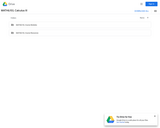Unrestricted Use
CC BY
Rating
0.0 stars

This contemporary calculus course is the third in a three-part sequence. In this course students continue to explore the concepts, applications, and techniques of Calculus - the mathematics of change. Calculus has wide-spread application in science, economics and engineering, and is a foundation college course for further work in these areas. This is a required class for most science and mathematics majors.Login: guest_oclPassword: ocl

Subject:
Calculus
Mathematics
Material Type:
Full Course
Homework/Assignment
Lecture Notes
Syllabus
Provider:
Washington State Board for Community & Technical Colleges
Provider Set:
Open Course Library
10/31/2011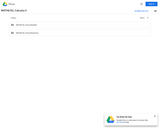Unrestricted Use
CC BY
Rating
0.0 stars

This contemporary calculus course is the second in a three-part sequence. In this course students continue to explore the concepts, applications, and techniques of Calculus - the mathematics of change. Calculus has wide-spread application in science, economics and engineering, and is a foundation college course for further work in these areas. This is a required class for most science and mathematics majors.Login: guest_oclPassword: ocl

Subject:
Calculus
Mathematics
Material Type:
Full Course
Homework/Assignment
Lecture Notes
Syllabus
Provider:
Washington State Board for Community & Technical Colleges
Provider Set:
Open Course Library
10/31/2011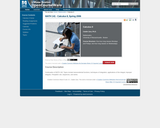Conditional Remix & Share Permitted
CC BY-NC-SA
Rating
0.0 stars

Topics in this course include transcendental functions, techniques of integration, applications of the integral, improper integrals, l'Hospital's rule, sequences, and series.

Subject:
Calculus
Mathematics
Material Type:
Full Course
Homework/Assignment
Syllabus
Provider:
UMass Boston
Provider Set:
UMass Boston OpenCourseWare
Author:
Catalin Zara
Ph.D.
04/25/2019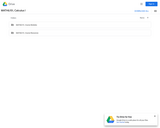Unrestricted Use
CC BY
Rating
0.0 stars

This course is an introduction to contemporary calculus and is the first of a three-part sequence. In this course students explore the concepts, applications, and techniques of Calculus - the mathematics of change. Calculus has wide-spread application in science, economics and engineering, and is a foundation college course for further work in these areas. This is a required class for most science and mathematics majors.Login: guest_oclPassword: ocl

Subject:
Calculus
Functions
Mathematics
Material Type:
Full Course
Homework/Assignment
Lecture Notes
Syllabus
Provider:
Washington State Board for Community & Technical Colleges
Provider Set:
Open Course Library
10/31/2011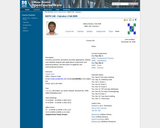Conditional Remix & Share Permitted
CC BY-NC-SA
Rating
0.0 stars

This course is an introduction to differential and integral calculus. It begins with a short review of basic concepts surrounding the notion of a function. Then it introduces the important concept of the limit of a function, and use it to study continuity and the tangent problem. The solution to the tangent problem leads to the study of derivatives and their applications. Then it considers the area problem and its solution, the definite integral. The course concludes with the calculus of elementary transcendental functions.

Subject:
Calculus
Mathematics
Material Type:
Full Course
Homework/Assignment
Syllabus
Provider:
UMass Boston
Provider Set:
UMass Boston OpenCourseWare
Author:
Catalin Zara
04/25/2019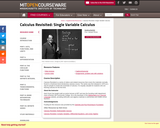Conditional Remix & Share Permitted
CC BY-NC-SA
Rating
0.0 stars

Calculus Revisited is a series of videos and related resources that covers the materials normally found in a freshman-level introductory calculus course.&nbsp; The series was first released in 1970 as a way for people to review the essentials of calculus.&nbsp; It is equally valuable for students who are learning calculus for the first time.

Subject:
Calculus
Mathematics
Material Type:
Full Course
Provider:
M.I.T.
Provider Set:
M.I.T. OpenCourseWare
Author:
Gross, Herbert
11/18/2012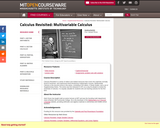Conditional Remix & Share Permitted
CC BY-NC-SA
Rating
0.0 stars

Calculus Revisited is a series of videos and related resources that covers the materials normally found in freshman- and sophomore-level introductory mathematics courses. Multivariable Calculus is the second course in the series, consisting of 26 videos, 4 Study Guides, and a set of Supplementary Notes. The series was first released in 1971 as a way for people to review the essentials of calculus. It is equally valuable for students who are learning calculus for the first time.

Subject:
Calculus
Mathematics
Material Type:
Full Course
Provider:
M.I.T.
Provider Set:
M.I.T. OpenCourseWare
Author:
Herbert Gross
01/01/2011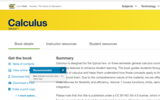Conditional Remix & Share Permitted
CC BY-NC-SA
Rating
0.0 stars

Calculus is designed for the typical two- or three-semester general calculus course, incorporating innovative features to enhance student learning. The book guides students through the core concepts of calculus and helps them understand how those concepts apply to their lives and the world around them. Due to the comprehensive nature of the material, we are offering the book in three volumes for flexibility and efficiency. Volume 1 covers functions, limits, derivatives, and integration.

Chapter 1: Functions and Graphs
Chapter 2: Limits
Chapter 3: Derivatives
Chapter 4: Applications of Derivatives
Chapter 5: Integration
Chapter 6: Applications of Integrations

Also available here: https://openstax.org/details/books/calculus-volume-1

Subject:
Calculus
Mathematics
Material Type:
Textbook
Provider:
Rice University
Provider Set:
OpenStax College
Author:
Alfred K. Mulzet
Catherine Abbott
David McCune
David Smith
David Torain
Edwin Jed Herman
Elaine A. Terry
Erica M. Rutter
Gilbert Strang
Joseph Lakey
Joyati Debnath
Julie Levandosky
Kirsten R. Messer
Michelle Merriweather
Nicoleta Virginia Bila
Sheri J. Boyd
Valeree Falduto
02/01/2016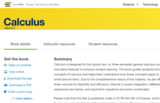Conditional Remix & Share Permitted
CC BY-NC-SA
Rating
0.0 stars

Calculus is designed for the typical two- or three-semester general calculus course, incorporating innovative features to enhance student learning. The book guides students through the core concepts of calculus and helps them understand how those concepts apply to their lives and the world around them. Due to the comprehensive nature of the material, we are offering the book in three volumes for flexibility and efficiency. Volume 2 covers integration, differential equations, sequences and series, and parametric equations and polar coordinates.

Access also available here: https://openstax.org/details/books/calculus-volume-2

Chapter 1: Integration

1.1 Approximating Areas
1.2 The Definite Integral
1.3 The Fundamental Theorem of Calculus
1.4 Integration Formulas and the Net Change Theorem
1.5 Substitution
1.6 Integrals Involving Exponential and Logarithmic Functions
1.7 Integrals Resulting in Inverse Trigonometric Functions
Chapter 2: Applications of Integration

2.1 Areas between Curves
2.2 Determining Volumes by Slicing
2.3 Volumes of Revolution: Cylindrical Shells
2.4 Arc Length of a Curve and Surface Area
2.5 Physical Applications
2.6 Moments and Centers of Mass
2.7 Integrals, Exponential Functions, and Logarithms
2.8 Exponential Growth and Decay
2.9 Calculus of the Hyperbolic Functions
Chapter 3: Techniques of Integration

3.1 Integration by Parts
3.2 Trigonometric Integrals
3.3 Trigonometric Substitution
3.4 Partial Fractions
3.5 Other Strategies for Integration
3.6 Numerical Integration
3.7 Improper Integrals
Chapter 4: Introduction to Differential Equations

4.1 Basics of Differential Equations
4.2 Direction Fields and Numerical Methods
4.3 Separable Equations
4.4 The Logistic Equation
4.5 First-order Linear Equations
Chapter 5: Sequences and Series

5.1 Sequences
5.2 Infinite Series
5.3 The Divergence and Integral Tests
5.4 Comparison Tests
5.5 Alternating Series
5.6 Ratio and Root Tests
Chapter 6: Power Series

6.1 Power Series and Functions
6.2 Properties of Power Series
6.3 Taylor and Maclaurin Series
6.4 Working with Taylor Series
Chapter 7: Parametric Equations and Polar Coordinates

7.1 Parametric Equations
7.2 Calculus of Parametric Curves
7.3 Polar Coordinates
7.4 Area and Arc Length in Polar Coordinates
7.5 Conic Sections

Subject:
Calculus
Mathematics
Material Type:
Textbook
Provider:
Rice University
Provider Set:
OpenStax College
Author:
Alfred K. Mulzet
Catherine Abbott
David McCune
David Smith
David Torain
Edwin Jed Herman
Elaine A. Terry
Erica M. Rutter
Gilbert Strang
Joseph Lakey
Joyati Debnath
Julie Levandosky
Kirsten R. Messer
Michelle Merriweather
Nicoleta Virginia Bila
Sheri J. Boyd
Valeree Falduto
02/01/2016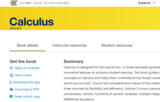Conditional Remix & Share Permitted
CC BY-NC-SA
Rating
0.0 stars

Calculus is designed for the typical two- or three-semester general calculus course, incorporating innovative features to enhance student learning. The book guides students through the core concepts of calculus and helps them understand how those concepts apply to their lives and the world around them. Due to the comprehensive nature of the material, we are offering the book in three volumes for flexibility and efficiency. Volume 3 covers parametric equations and polar coordinates, vectors, functions of several variables, multiple integration, and second-order differential equations.

Access also available here: https://openstax.org/details/books/calculus-volume-3

1. Parametric Equations and Polar Coordinates

1.1. Introduction
1.2. Parametric Equations
1.3. Calculus of Parametric Curves
1.4. Polar Coordinates
1.5. Area and Arc Length in Polar Coordinates
1.6. Conic Sections
2. Vectors in Space

2.1. Introduction
2.2. Vectors in the Plane
2.3. Vectors in Three Dimensions
2.4. The Dot Product
2.5. The Cross Product
2.6. Equations of Lines and Planes in Space
2.8. Cylindrical and Spherical Coordinates
3. Vector-Valued Functions

3.1. Introduction
3.2. Vector-Valued Functions and Space Curves
3.3. Calculus of Vector-Valued Functions
3.4. Arc Length and Curvature
3.5. Motion in Space
4. Differentiation of Functions of Several Variables

4.1. Introduction
4.2. Functions of Several Variables
4.3. Limits and Continuity
4.4. Partial Derivatives
4.5. Tangent Planes and Linear Approximations
4.6. The Chain Rule
4.7. Directional Derivatives and the Gradient
4.8. Maxima/Minima Problems
4.9. Lagrange Multipliers
5. Multiple Integration

5.1. Introduction
5.2. Double Integrals over Rectangular Regions
5.3. Double Integrals over General Regions
5.4. Double Integrals in Polar Coordinates
5.5. Triple Integrals
5.6. Triple Integrals in Cylindrical and Spherical Coordinates
5.7. Calculating Centers of Mass and Moments of Inertia
5.8. Change of Variables in Multiple Integrals
6. Vector Calculus

6.1. Introduction
6.2. Vector Fields
6.3. Line Integrals
6.4. Conservative Vector Fields
6.5. Green's Theorem
6.6. Divergence and Curl
6.7. Surface Integrals
6.8. Stokes' Theorem
6.9. The Divergence Theorem
7. Second-Order Differential Equations

7.1. Introduction
7.2. Second-Order Linear Equations
7.3. Nonhomogeneous Linear Equations
7.4. Applications
7.5. Series Solutions of Differential Equations
Table of Integrals
Table of Derivatives
Review of Pre-Calculus

Subject:
Calculus
Mathematics
Material Type:
Textbook
Provider:
Rice University
Provider Set:
OpenStax College
Author:
Alfred K. Mulzet
Catherine Abbott
David McCune
David Smith
David Torain
Edwin Jed Herman
Elaine A. Terry
Erica M. Rutter
Gilbert Strang
Joseph Lakey
Joyati Debnath
Julie Levandosky
Kirsten R. Messer
Michelle Merriweather
Nicoleta Virginia Bila
Sheri J. Boyd
Valeree Falduto
02/01/2016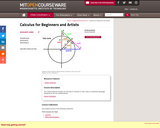Conditional Remix & Share Permitted
CC BY-NC-SA
Rating
0.0 stars

This online textbook provides an overview of Calculus in clear, easy to understand language designed for the non-mathematician.

Subject:
Calculus
Mathematics
Material Type:
Full Course
Provider:
M.I.T.
Provider Set:
M.I.T. OpenCourseWare
Author:
Kleitman, Daniel
01/01/2005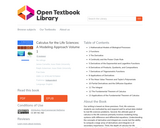Conditional Remix & Share Permitted
CC BY-NC-SA
Rating
0.0 stars

Our writing is based on three premises. First, life sciences students are motivated by and respond well to actual data related to real life sciences problems. Second, the ultimate goal of calculus in the life sciences primarily involves modeling living systems with difference and differential equations. Understanding the concepts of derivative and integral are crucial, but the ability to compute a large array of derivatives and integrals is of secondary importance. Third, the depth of calculus for life sciences students should be comparable to that of the traditional physics and engineering calculus course; else life sciences students will be short changed and their faculty will advise them to take the 'best' (engineering) course.

Subject:
Calculus
Mathematics
Material Type:
Textbook
Provider:
University of Minnesota
Provider Set:
University of Minnesota Libraries Publishing
Author:
James L. Cornette
Ralph A. Ackerman
01/01/2012Conditional Remix & Share Permitted
CC BY-NC-SA
Rating
0.0 stars

This books covers the following topics: Geometry of Rn; functions from R to Rn; functions from Rn to R; functions from Rm to Rn.

Subject:
Calculus
Mathematics
Material Type:
Homework/Assignment
Textbook
Provider:
Furman University
Author:
Dan Sloughter
04/25/2019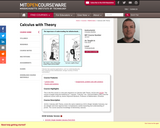Conditional Remix & Share Permitted
CC BY-NC-SA
Rating
0.0 stars

Calculus with Theory, covers the same material as 18.01 (Single Variable Calculus), but at a deeper and more rigorous level. It emphasizes careful reasoning and understanding of proofs. The course assumes knowledge of elementary calculus.

Subject:
Calculus
Mathematics
Material Type:
Full Course
Provider:
M.I.T.
Provider Set:
M.I.T. OpenCourseWare
Author:
Breiner, Christine
01/01/2010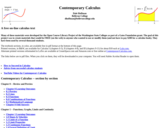Unrestricted Use
CC BY
Rating
0.0 stars

Chapter 0 -- Review and Preview
Chapter 1 -- Functions, Graphs, Limits and Continuity
Chapter 2 -- The Derivative
Chapter 3 -- Derivatives and Graphs
Chapter 4 -- The Integral
Chapter 5 -- Applications of Definite Integrals
Chapter 6 -- Introduction to Differential Equations
Chapter 7 -- Inverse Trigonometric Functions
Chapter 8 -- Improper Integrals and Integration Techniques
Chapter 9 -- Polar, Parametric & Conics
Chapter 10 -- Infinite Series & Power Series
Chapter 11 -- Vectors, Lines and Planes in 3D
Chapter 12 -- Vector-Valued Functions
Chapter 13 -- Functions of Several Variables
Chapter 14 -- Double and Triple Integrals
Chapter 15 -- Vector Calculus

Subject:
Calculus
Mathematics
Material Type:
Textbook
Author:
Dale Hoffman
11/30/2020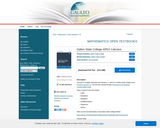Conditional Remix & Share Permitted
CC BY-NC-SA
Rating
0.0 stars

This text for Analytic Geometry and Calculus I, II, and III is a Dalton State College remix of APEX Calculus 3.0.

Subject:
Calculus
Mathematics
Material Type:
Textbook
Author:
Jason Schmurr
Michael Hilgemann
Thomas Gonzalez
03/28/2019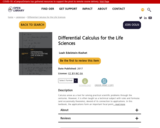Conditional Remix & Share Permitted
CC BY-NC-SA
Rating
0.0 stars

Calculus arose as a tool for solving practical scientific problems through the centuries. However, it is often taught as a technical subject with rules and formulas (and occasionally theorems), devoid of its connection to applications. In this textbook, the applications form an important focal point, with emphasis on life sciences. This places the techniques and concepts into practical context, as well as motivating quantitative approaches to biology taught to undergraduates. While many of the examples have a biological flavour, the level of biology needed to understand those examples is kept at a minimum. The problems are motivated with enough detail to follow the assumptions, but are simplified for the purpose of pedagogy.

1 Power functions as building blocks
2 Average rates of change, average velocity and the secant line
3 Three faces of the derivative: geometric, analytic, and computational
4 Differentiation rules, simple antiderivatives and applications
5 Tangent lines, linear approximation, and Newton’s method
6 Sketching the graph of a function using calculus tools
7 Optimization
8 Introducing the chain rule
9 Chain rule applied to related rates and implicit differentiation
10 Exponential functions
11 Differential equations for exponential growth and decay
12 Solving differential equations
13 Qualitative methods for differential equations
14 Periodic and trigonometric functions
15 Cycles, periods, and rates of change

Subject:
Calculus
Mathematics
Material Type:
Textbook
Author:
Leah Edelstein-Keshet
11/30/2020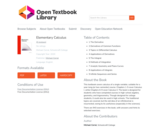Rating
0.0 stars

This textbook covers calculus of a single variable, suitable for a year-long (or two-semester) course. Chapters 1-5 cover Calculus I, while Chapters 6-9 cover Calculus II. The book is designed for students who have completed courses in high-school algebra, geometry, and trigonometry. Though designed for college students, it could also be used in high schools. The traditional topics are covered, but the old idea of an infinitesimal is resurrected, owing to its usefulness (especially in the sciences).

There are 943 exercises in the book, with answers and hints to selected exercises.

1 The Derivative
2 Derivatives of Common Functions
3 Topics in Differential Calculus
4 Applications of Derivatives
5 The Integral
6 Methods of Integration
7 Analytic Geometry and Plane Curves
8 Applications of Integrals
9 Infinite Sequences and Series

Subject:
Calculus
Mathematics
Material Type:
Textbook
Author:
Michael Corral
01/20/2021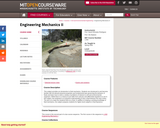Conditional Remix & Share Permitted
CC BY-NC-SA
Rating
0.0 stars

This subject provides an introduction to fluid mechanics. Students are introduced to and become familiar with all relevant physical properties and fundamental laws governing the behavior of fluids and learn how to solve a variety of problems of interest to civil and environmental engineers. While there is a chance to put skills from Calculus and Differential Equations to use in this subject, the emphasis is on physical understanding of why a fluid behaves the way it does. The aim is to make the students think as a fluid. In addition to relating a working knowledge of fluid mechanics, the subject prepares students for higher-level subjects in fluid dynamics.

Subject:
Applied Science
Calculus
Environmental Science
Mathematics
Material Type:
Full Course
Provider:
M.I.T.
Provider Set:
M.I.T. OpenCourseWare
Author:
01/01/2006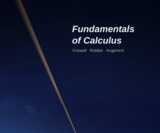Conditional Remix & Share Permitted
CC BY-SA
Rating
0.0 stars

This open-source book by Crowell, Robbin, and Angenent is a spin-off of a previous open-source book by Robbin and Angenent. It covers the first semester of a freshman calculus course.

Subject:
Calculus
Mathematics
Material Type:
Textbook
Provider:
Light and Matter
Provider Set:
Light and Matter Books
Author:
Crowell
Robin and Angenent